## Complete Table of Example Orbits

Here we focus on two values of a, namely the extreme case a = M and a non-extreme case a = 0.5M. Phi takes the range -7M < Phi < 2M in the former case, while it takes the range -6.1382M < Phi < 4.0963M in the latter case. Example orbits are chosen at more or less equal intervals along these ranges.

In most of the examples, the orbits have been plotted for an integer number of latitudinal oscillations until they return to near the starting point. Again, clicking on each figure gives a three-dimensional wire-frame model of the orbit.

a = M a = 0.5M
Phi = -6.9M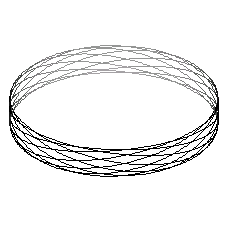Phi = -6M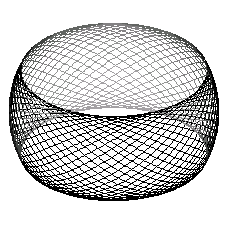Phi = -5MPhi = -4M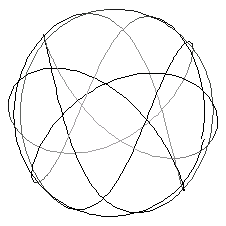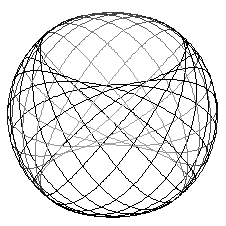Phi = -3MPhi = -2M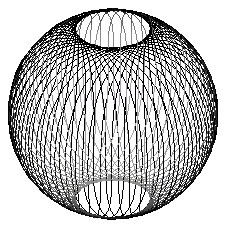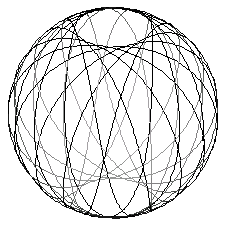Phi = -M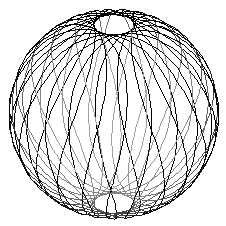Phi = 0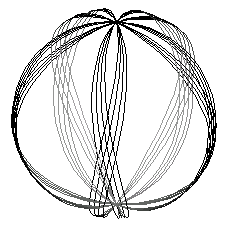Phi = MPhi = 1.9M
(for a = M)

Phi = 2M
(for a = 0.5M)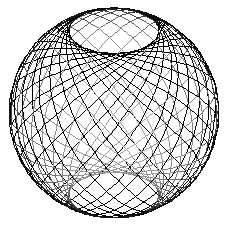Phi = 3M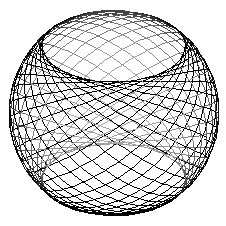Phi = 4M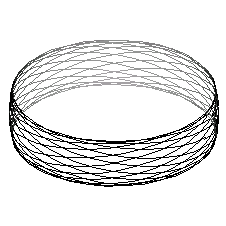## Back to Main Page

by Edward Teo [Home]